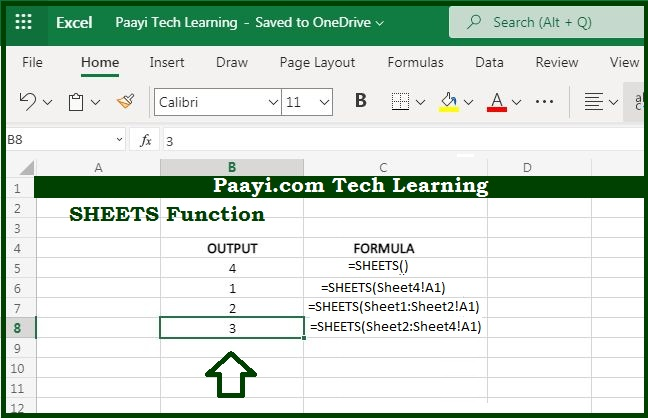# Learn How to Use Microsoft Excel SHEETS Function

Written by | 0 Comments | 650 Views

In this article, you will learn how to use the Microsoft Excel SHEETS function and its prime function in Microsoft Excel. You will also get to know the Microsoft Excel SHEETS function return value and syntax with the help of some examples.

Microsoft Excel SHEETS Function

The main use of the Microsoft Excel "SHEETS" Function is to get the number of sheets in a reference. So, you can able to return the total number of sheets in a given reference. In case the argument is not provided, the SHEETS function returns the total number of sheets in the workbook.

Return Value of SHEETS Function

The return value will be the sheet count.

Syntax of SHEETS Function

=SHEETS([reference] )

Where the arguments:

value: It is the valid reference (optional).

## How to Use Microsoft Excel SHEETS Function?The main use of the Microsoft Excel "SHEETS" Function is to get the number of sheets in a reference. So, you can able to return the total number of sheets in a given reference. In case the argument is not provided, the SHEETS function returns the total number of sheets in the workbook.

It should be noted that:

The SHEET function includes hidden sheets in the count.

In case the value argument is not present, the SHEETS function will return the total sheet count.

The SHEET function reports the index of a sheet, the SHEETS function reports the number of sheets in a reference.

The SHEETS function will report the sheet count in 3D reference.